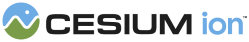#CubicRealPolynomial

Defines functions for 3rd order polynomial functions of one variable with only real coefficients.

### Methods

#### static Cesium.CubicRealPolynomial.computeDiscriminant(a, b, c, d) → Number Core/CubicRealPolynomial.js 20

Provides the discriminant of the cubic equation from the supplied coefficients.
Name Type Description
`a` Number The coefficient of the 3rd order monomial.
`b` Number The coefficient of the 2nd order monomial.
`c` Number The coefficient of the 1st order monomial.
`d` Number The coefficient of the 0th order monomial.
##### Returns:
The value of the discriminant.

#### static Cesium.CubicRealPolynomial.computeRealRoots(a, b, c, d) → Array.<Number> Core/CubicRealPolynomial.js 164

Provides the real valued roots of the cubic polynomial with the provided coefficients.
Name Type Description
`a` Number The coefficient of the 3rd order monomial.
`b` Number The coefficient of the 2nd order monomial.
`c` Number The coefficient of the 1st order monomial.
`d` Number The coefficient of the 0th order monomial.
##### Returns:
The real valued roots.
Need help? The fastest way to get answers is from the community and team on the Cesium Forum.